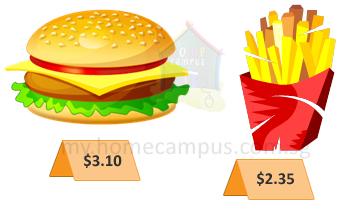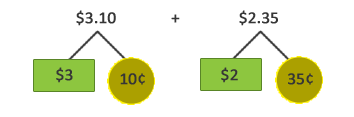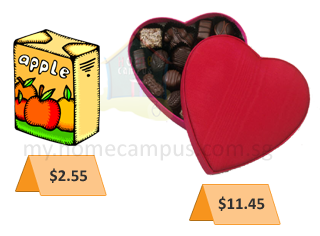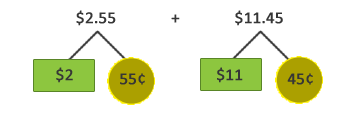#### 1. Carol bought a burger. She also bought some fries. How much did she spend altogether?10¢  +  35¢  =  45¢

\$3  +  \$2  =  \$5

Step 3: Add the dollars and cents.
\$5  +  45¢  =  \$5.45

She spent \$5.45 altogether.

#### 2. A carton of juice costs \$2.55. A box of chocolates costs \$11.45. What is the total cost of the carton of juice and the box of chocolates?55¢  +  45¢  =  100¢ =  \$1

\$2  +  \$11  =  \$13

Step 3:
\$2.55  +  \$11.45  =  \$13  +  \$1 =  \$14

The total cost of the carton of juice and the box of chocolates is \$14.

#### 3. Add \$12.35 and \$9.75.

\$12.35  +  \$9.75  =  ?